Test: Instruction Pipeline

# Test: Instruction Pipeline - Electronics and Communication Engineering (ECE)

Test Description

## 10 Questions MCQ Test Topicwise Question Bank for Electronics Engineering - Test: Instruction Pipeline

Test: Instruction Pipeline for Electronics and Communication Engineering (ECE) 2023 is part of Topicwise Question Bank for Electronics Engineering preparation. The Test: Instruction Pipeline questions and answers have been prepared according to the Electronics and Communication Engineering (ECE) exam syllabus.The Test: Instruction Pipeline MCQs are made for Electronics and Communication Engineering (ECE) 2023 Exam. Find important definitions, questions, notes, meanings, examples, exercises, MCQs and online tests for Test: Instruction Pipeline below.
Solutions of Test: Instruction Pipeline questions in English are available as part of our Topicwise Question Bank for Electronics Engineering for Electronics and Communication Engineering (ECE) & Test: Instruction Pipeline solutions in Hindi for Topicwise Question Bank for Electronics Engineering course. Download more important topics, notes, lectures and mock test series for Electronics and Communication Engineering (ECE) Exam by signing up for free. Attempt Test: Instruction Pipeline | 10 questions in 30 minutes | Mock test for Electronics and Communication Engineering (ECE) preparation | Free important questions MCQ to study Topicwise Question Bank for Electronics Engineering for Electronics and Communication Engineering (ECE) Exam | Download free PDF with solutions
 1 Crore+ students have signed up on EduRev. Have you?
*Multiple options can be correct
Test: Instruction Pipeline - Question 1

### Consider a 5-stage pipeline having stages as Instruction Fetch (IF), Instruction Decode (ID), Operand Fetch (OF), Execute (EX) and Write Back (WB). Here we are given 4 instructions. IF, ID, OF and WB stages take 1 clock cycle each, but the EX-stage takes 1 cycle for ADD and SUB, 2 cycles for MUL and 3 cycles for DIV operation. If the operand forwarding technique is used from EX stage to OF stage and the clock rate of pipeline processor is 5 GHz, then choose the correct statement(s), considering the below table: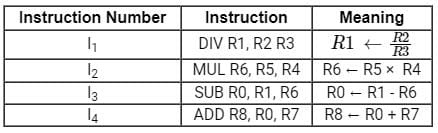Detailed Solution for Test: Instruction Pipeline - Question 1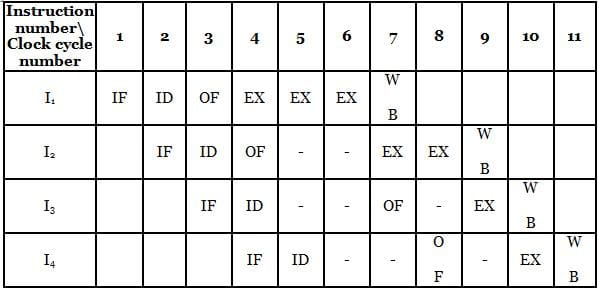Here operand forwarding is used from EX to OF stage.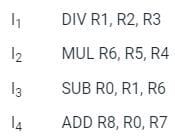Instruction I3 is dependent on both I1 and I2, so we will fetch the operand for I3 after execution of instruction I1.
When execution of I2 gets completed, we can execute the instruction I3.
Similarly, I4 is dependent on I3. We can only execute I4 after the execution of I3.
Option (1)- True, from above diagrammatic representation, we can see that I3 gets executed in 10th cycle.
Option (2)- True, 3 RAW (Read After Write) dependencies are there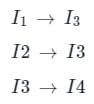So, total RAW dependencies are 3.
RAW is also known as True dependency.
Option (3)- True, Last instruction I4 gets executed in 11th cycle.
So, total clock cycles required are 11.
Option (3)- True, Total execution time = Total number of clock cycles × clock cycle time
Clock cycle time = 1/Clock rate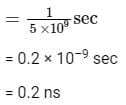Total execution time = 11 × 0.2 ns
= 2.2 ns
= 2.2 x 10–9 sec
= 2.2 x 103 × 10–12 sec
= 2200 x 10–12 sec
= 2200 picoseconds

Test: Instruction Pipeline - Question 2

### In microprocessors, the IC (instruction cycle), FC (fetch cycle) and EC (execution cycle) are related as

Detailed Solution for Test: Instruction Pipeline - Question 2
• The Steps required by the CPU to fetch and execute an Instruction is called an instruction cycle. It consists of fetch and executes cycle.
• Instruction cycle (IC) = Fetch cycle (FC) + Execution cycle (EC)
• The time required by the microprocessor to complete the operation of accessing memory or I/O devices is called a machine cycle.
• Clock time is a known time state. It is reciprocal of clock frequency.
• Instruction cycle > Machine cycle > Clock cycle (time state)
Test: Instruction Pipeline - Question 3

### A non-pipelined CPU has 12 general purpose registers (R0, R1, R2,….R12). Following operations are supported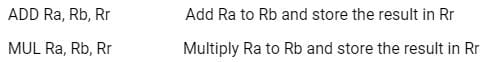MUL operations takes two clock cycles, ADD takes one clock cycle. Calculate minimum number of clock cycles required to compute the value of the expression XY + XYZ + YZ. The variables X, Y, Z are initially available in registers R0, R1 and R2 and contents of these registers must not be modified.

Detailed Solution for Test: Instruction Pipeline - Question 3

XY + XYZ + YZ = (X × Y) + (X × Y × Z) + (Y × Z) = (X × Y) + (X × Y + Y) × Z
The instructions are non-pipelined and cycles for each instruction is mentioned. Therefore,
X × Y - takes 2 cycles
X × Y + Y - takes 1 cycles (X × Y already done)
(X × Y + Y) × Z - takes 2 cycles
(X × Y) + (X × Y + Y) × Z - takes 1 cycle
Hence, total cycles = 2 + 1 + 2 + 1 = 6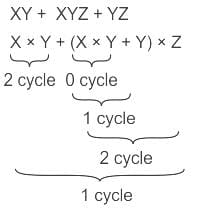*Answer can only contain numeric values
Test: Instruction Pipeline - Question 4

Consider a non-pipelined processor operating at 2.5 GHz. It takes 5 clock cycles to complete an instruction. You are going to make a 5-stage pipeline out of this processor. Overheads associated with pipelining force you to operate the pipelined processor at 2 GHz. In a given program, assume that 30% are memory instructions, 60% are ALU instructions and the rest are branch instructions. 5% of the memory instructions cause stalls of 50 clock cycles each due to cache misses and 50% of the branch instructions cause stalls of 2 cycles each. Assume that there are no stalls associated with the execution of ALU instructions. For this program, the speedup achieved by the pipelined processor over the non-pipelined processor (round off to 2 decimal places) is _____.

Detailed Solution for Test: Instruction Pipeline - Question 4

Data:

For a non-pipelined processor,
Clock cycles to complete one instruction = 5
Instruction operating frequency = 2.5 GHz
One clock cycle time = 1/ (2.5 GHz) = 0.4 ns
For N number of instructions, clock cycles required = 5N
Time taken to complete 5n clock cycles = 0.4*5n = 2N ns
For a pipelined processor,
Stages of pipeline = 5
One clock cycle time = 1/ (2 GHz) = 0.5 ns
For n instructions, clock cycles required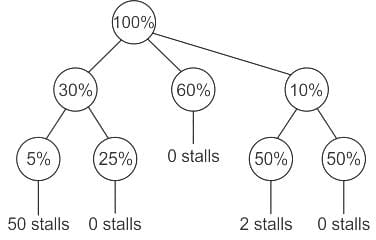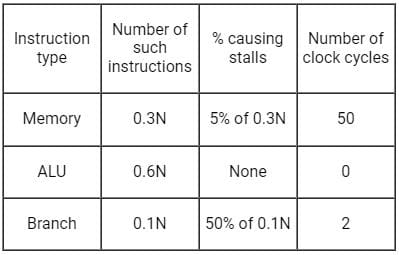Therefore, time taken by pipelined processor:
0.6N (1) + 0.3N [0.05 (1 + 50) + 0.95 (1)] + 0.1N [0.5 (1 + 2) + 0.5 (1)] cycles
= 1.85N cycles
= 1.85N/2 ns
= 0.925N ns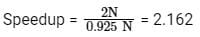Test: Instruction Pipeline - Question 5

A non-pipeline system takes 50ns to process a task. The same task can be processed in six-segment pipeline with a clockcycle of 10ns. Determine approximately the speedup ratio of the pipeline for 500 tasks.

Detailed Solution for Test: Instruction Pipeline - Question 5

Data:
Time for non-pipelined execution per task = tn = 50 ns
Time for pipelined execution per task =  tp = 10 ns
Number of stages in the pipeline = k = 6
Formula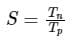S = speed up factor

Calculation:
Time for non-pipelined = Tn = tn x Number of tasks
Time for non-pipelined = Tn =  50 x 500
Time for pipelined = Tp​ = 1st task x k x tp + (All Remaining Tasks (k - 1)) x tp
Time for pipelined = Tp​ =  1 x 6 x 10 + (500 - 1) x 10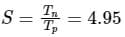Test: Instruction Pipeline - Question 6

The first machine cycle of an instruction is always a

Detailed Solution for Test: Instruction Pipeline - Question 6

Machine Cycle: Time taken to execute one OPERATION is known as a machine cycle.  One instruction will contain 1 to 5 machine cycles.
T-State: The portion of a machine cycle executed in one internal clock pulse is known as T-state.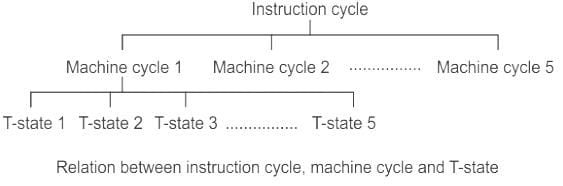Steps in the instruction cycle:

• First of all, the opcode is fetched by the microprocessor from a stored memory location.
• Then it is decoded by the microprocessor to find out which operation it needs to perform.
• If an instruction contains data or operand address which is still in the memory, the CPU has to perform read operation to get the desired data.
• After receiving the data, it performs to execute the operation.

Test: Instruction Pipeline - Question 7

Which one of the following is false about Pipelining?

Detailed Solution for Test: Instruction Pipeline - Question 7

In pipelining, each step operates parallel with other steps. It stores and executes instructions in an orderly manner.
The main advantages of using pipeline are :

• It increases the overall instruction throughput.
• Pipeline is divided into stages and stages are connected to form a pipe-like structure.
• We can execute multiple instructions simultaneously.
• It makes the system reliable.
• It increases the program speed.
• It reduces the overall execution time but does not reduce the individual instruction time.

​Therefore option 2 is the false statement about Pipelining

Test: Instruction Pipeline - Question 8

Consider the following table: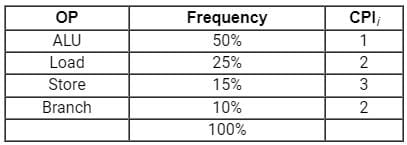Which of the following is true about the average CPI of the above given table?

Detailed Solution for Test: Instruction Pipeline - Question 8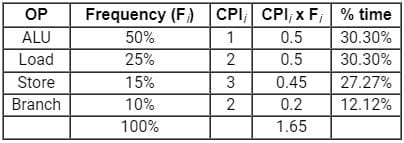• ALU takes ~30.30% of the total cycles.
• Load takes ~30.30% of the total cycles.
• Store takes ~27.27% of total cycles.
• Branch takes ~12.12% of total cycles.
Test: Instruction Pipeline - Question 9

Pipelining increases ______ of the processor.

Detailed Solution for Test: Instruction Pipeline - Question 9

In pipelining, each step operates parallel with other steps. It stores and executes instructions in an orderly manner.
The main advantages of using pipeline are :

• It increases the overall instruction throughput.
• Pipeline is divided into stages and stages are connected to form a pipe-like structure.
• We can execute multiple instructions simultaneously.
• It makes the system reliable.
• It increases the program speed.
• It reduces the overall execution time but does not reduce the individual instruction time.
Test: Instruction Pipeline - Question 10

Consider the following sequence of micro-operations.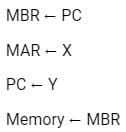Which one of the following is a possible operation performed by this sequence?

Detailed Solution for Test: Instruction Pipeline - Question 10
1. Program counter holds the next instruction value to be executed.
Here, MBR <- PC means the value of the program counter will get stored in MBR.
2. MAR <- X means some address value X is storing in MAR so to access memory location X.
3. PC <- Y means storing new instruction value Y to the program counter to access new instruction.
4. Memory <- MBR means MBR register will store its value to Memory. This saves the previous value of PC to memory.

This sequence of instructions matches with Interrupt Service Routine (ISR) since the sequence of instructions saved the address of current instructions into memory.
Hence, the correct answer is “option 4”.

## Topicwise Question Bank for Electronics Engineering

193 tests
Information about Test: Instruction Pipeline Page
In this test you can find the Exam questions for Test: Instruction Pipeline solved & explained in the simplest way possible. Besides giving Questions and answers for Test: Instruction Pipeline, EduRev gives you an ample number of Online tests for practice

## Topicwise Question Bank for Electronics Engineering

193 tests(Scan QR code)